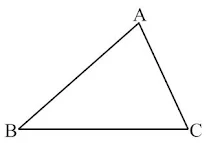# Chapter 6 The Triangle and its Properties Class 7 Notes Maths

Chapter 6 The Triangle and its Properties Class 7 Notes Maths is given here will provide a quick glimpse of the chapter and improve the learning experience. It will make you aware of the all the topics and ensure consistency in the preparation level. Also, NCERT Solutions for Class 7 Chapter 6 Maths that will help in completing homework on time. You will understand the various factors through which one can improve their efficiency and eventually scores higher in the exam. It is quite easy to retain the answers once you are fully aware of the concept thus Class 7 Revision Notes can be beneficial for you.Introduction

• A triangle is a simple closed curve made of three line segments. It has three vertices, three sides and three angles.Angles: ∠BAC, ∠ABC, ∠BCA
Vertices: A, B, C

Types of Triangles based on Sides

(i) Equilateral triangle: A triangle having all sides equal, is called an equilateral triangle.
(ii) Isosceles triangle: A triangle having two sides equal, is called an isosceles triangle.
(iii) Scalene triangle: A triangle having all sides of different lengths is called a scalene triangle.

Types of Triangles based on Angles

(i) Acute triangle: A triangle each of whose angle measures less than 90° is called an acute triangle.
(ii) Right angled triangle: A triangle one of whose angle measures 90° is called a right angled triangle.
(iii) Obtuse triangle: A triangle one of whose angle measures more than 90° is called an obtuse triangle.

• A median of a triangle connects a vertex of a triangle to the mid-point of the opposite side.

• An altitude of a triangle has one end point at a vertex of the triangle and the other on the line containing the opposite side. Through each vertex, an altitude can be drawn.

Properties of a Triangle

Property of Exterior Angles

• The measure of any exterior angle of a triangle is equal to the sum of the measures of its interior opposite angles.

Angle sum property

• The sum of three angles of a triangle is 180°.

Property of the Lengths of Sides of a Triangle

• The sum of the lengths of any two sides of a triangle is always greater than the length of the third side.

• The difference of the lengths of any two sides of a triangle is always smaller than the length of the third side.

Pythagoras Property

• In a right-angled triangle, the side opposite to the right angle is called the hypotenuse and the other two sides are called its legs or arms (base and perpendicular).

• In a right-angled triangle, the square of the hypotenuse is equal to the sum of the squares of its legs.